## Tweaking Newton’s Law of Universal Gravitation

I answered a question on Quora about the consequences of Newton’s law of Universal Gravitation being in inverse law and not an inverse square law for 60 seconds. The outcome is not so good, what with all matter acting as a black hole and all.

#### What would happen if the law of gravity becomes ~1/r instead of ~1/r² for 60 seconds?

Colin Turner’s answer: All matter in the Universe would act as a black hole for 60 seconds. Which could be bad? You may recall that work done against a Force is given by WD = F.s I.e. Force times distance. In any varying force you would have to use the big daddy of multiplication : integratio…

## Black Hole Radius, or How I came to love the LHC

I allowed myself to become rather distracted by my second years last week as the class was finishing. They were talking about an episode of Horizon that discussed General Relativity and theories of Quantum Gravity. What followed was a free ranging discussion on the nature of infinity, mentioned briefly in the program. But we also talked about the nature of a black hole and its size.

It’s surprisingly easy to calculate this with reasonably elementary maths and physics. I first did this when I was about 17 (how very sad) using classical physics equations, and was astounded to discover that even so, the answer was correct (I checked it in the Encyclopedia Britannica in the library at the time).

Here is Newton’s universal law of gravitation, between two bodies. It describes the force F between two bodies that are r metres apart. Let’s take the one with mass M to be the black hole. G is a small (though mysterious) constant.You can work out the energy needed to escape the black hole using the old stand by equation that work done is the force times distance traveled against that force, but that only works with a constant force, this force will change as we move, so we need to use the big daddy of multiplication, integration.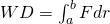Specifically, we will work out the energy needed to escape from the event horizon, the surface at which the escape velocity is the speed of light, which is c (299,792,458 m/s). So the energy will be given by moving my little mass m from the radius of the event horizon, let’s call is R to infinity, to show we have broken away.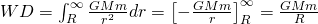Now, this should just balance the kinetic energy possessed by my little mass m traveling at the speed of light.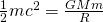and if we rearrange for R we get that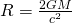In other words, the radius of the event horizon, the bit we think of as the “hole” is dependent entirely upon the mass of the object. Please note this is based on a very simple model of a non rotating black hole. Nevertheless we can do some nice calculations from this.

The Sun, would have to be compressed from its diameter of about 700 million kilometres into a radius of just under 3 kilometres. The Earth’s mass would need to be compressed so much to form a black hole you would need to squeeze its radius of over 6 thousand kilometres into a radius of around 9 millimetres. That’s how dense we’re talking here.

We can also consider the radius as described by the contained energy of the black hole, since we know that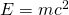and so, replacing our M in our above equation we get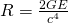Wow. Remember c is a big number, taking it to the power of four is a lot. So why do this? There’s been a lot of speculation about the possibility the Large Hadron Collider (LHC) could create a black hole. This has caused a fair degree of panic, and at least one suicide. It’s a physicist’s dream that a black hole might be created. I just looked up the “high” energies used by the LHC, and high is a relative term. It plans to bash protons together with 7 TeV (Tera electron volts) of energy each, or lead nucleii with 574 TeV each, let’s take the latter. Just how much energy is that in a collision? Well, doubling and converting to good old Joules gives 184 micro Joules. That’s really not a lot, 184 millionth’s of a Joule. A 100W light bulb uses 100 Joules each and every second. How big would the radius of such a black hole that might form be, from that energy? Check the maths, because so far I haven’t but I get.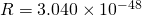metres

which is 0.000,000,000,000,000,000,000,000,000,000,000,000,000,000,000,003 metres wide (I hope, I didn’t double check the zeros either), which is probably not the planet swallower of people’s imagination (but this is fun). But the problem is people think this tiddler will grow very rapidly, but that’s because they don’t know about Hawking Radiation. This is an interesting quantum effect that means black holes aren’t really black, they do emit a little radiation. Large holes would gather surrounding matter faster than their low radiation rate, but small holes have the opposite situation, they radiate more rapidly. The maths for all that is pretty complex, and you need to make lots of assumptions, but the time taken for our little black hole to “evaporate” is (hurriedly calculated by me)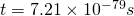a tiny, tiny fraction of a second. Even allowing for the ambient temperature and some fall in of matter, this little baby is not in equilibrium, it’s not getting mass fast enough to accumulate more. It’s safe*.

* All disclaimers apply. No liability is assumed for foolish unvalidated experiments done by you or other members of your species. Do not attempt to create black holes in your garage. Any subsequent destruction of your civilization, planet or solar system is at your own risk, and any “EPIC FAIL” signs placed by aliens on the remains is not due to me or my calculation. No calculations have been done on the matter of strange matter either. If you break the planet / system / galaxy or universe you own all the parts.